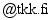[back to homepage]

# Geometric interpretation of the complex Riccati equation

#### Journal of Nonlinear Mathematical Physics, Vol. 14, Issue 1, pp. 95-111, 2007

##### Mathematica toolbooks:
• Eigenvalues of 4x4 matrix appearing in the proof of Proposition 2.2 [ .nb , .html ]
• Proof of identity for 2x2 matrices used Section 5.1: [ .nb , .html ]
• Plot Figure 1.: [ .nb , .html ]
• Section 5.2: Check that expressions for K(t) and S(t) are correct: [ .nb , .html ]
• Section 5.2: Check that K(t) and S(t) can be calculated either using Proposition 2.2 or from eigenvalues of \$G\$: [ .nb , .html ]
• Plot Figure 2.: [ .nb , .html ]
• Plot Figure 3.: [ .nb , .html ]
If you have questions or comments, please contact me at matias.dahl.